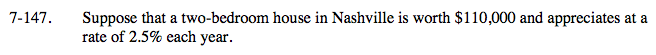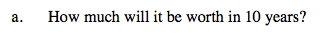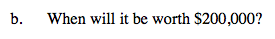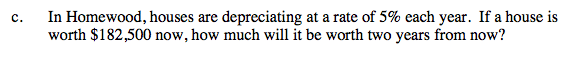### Home > CAAC > Chapter 13 > Lesson 13.OF2-S > Problem7-147

7-147.
1. Suppose that a two-bedroom house in Nashville is worth $110,000 and appreciates at a rate of 2.5% each year. Homework Help ✎ 1. How much will it be worth in 10 years? 2. When will it be worth$200,000?

3. In Homewood, houses are depreciating at a rate of 5% each year. If a house is worth \$182,500 now, how much will it be worth two years from now?General form: y = abx

Let x = number of years and y = value of house. Write an equation using the general form of the exponential equation.

a is the initial value of the house, and b is the multiplier.

y = 110,000(1.025)xUsing the equation in part (a), guess and check values for x. Your answer will be approximate.Write another equation. Note that the value is depreciating this time.